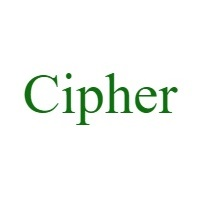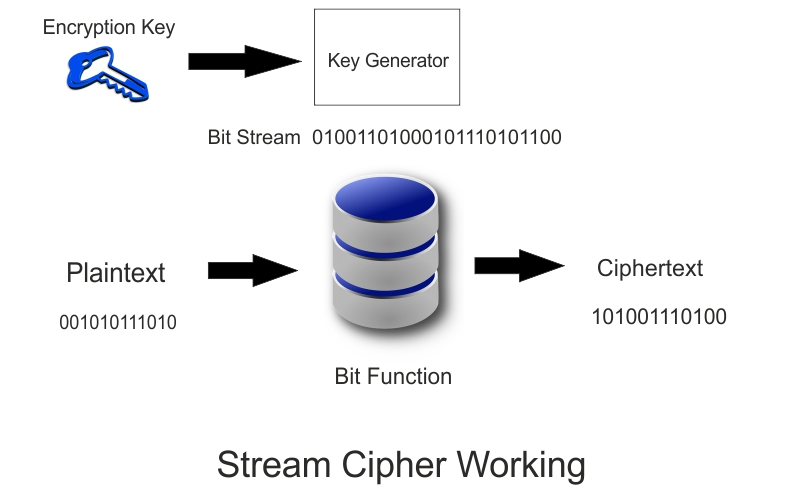# What Is A Cipher | Types Of Ciphers | Working Of Cipher## Introduction Of Cipher

Whenever we gets in touch of Cryptography then we gets touch in terms like Cipher, Cypher, Caesar Cipher, Ciphertext, Plaintext, Cipher System and Cipher Code. These are most common terms used in cryptography. Because Various logics and functions works in cryptography. Somehow there is a little bit of difference between these terms in cryptography.

So today in this article we will cover what is a cipher, Types Of Ciphers & Working with all of the above mentioned Cryptographic Terms. We will not make complicated this article but will explain each and every concept point to point in simple words.

## What Is A Cipher

Cipher is used to Encrypt the data using Encryption Algorithm with the help of Encryption Key. Where data is known as Plaintext and encrypted data is known as Ciphertext Cipher is also called as Cypher. Cypher is pronounced as SAI-FHUR. We can read Plaintext and we can not read Ciphertext because it is encrypted code.

A historical cipher is Caesar Cipher which used in military operations. Cipher System is also known as Cryptosystem.

Codes and Ciphers are used to perform the same task.  But the conceptual difference between Codes and Ciphers is that codes gives output string of different character length while ciphers gives same length of characters as of input.

## Types Of Ciphers & WorkingAs shown above in the figure first sender sends Plaintext in the encryption server. This encryption server uses encryption algorithm and encryption key to encrypt the Plainttext. After encryption this encryption server provide Ciphertext as encrypted output.

Now keep in mind one thing is that this interceptor can see the ciphertext and decryption algorithm. But can not have decryption key to see the plaintext which we send in it.

For the decryption process this Ciphertext goes into the decryption server where decryption algorithm is applied on Ciphertext with the decryption key. This decryption server provides Plaintext as decrypted output to the receiver.### There are two types of Ciphers mentioned below,

(1) Block Cipher

(2) Stream Cipher

### (1) Block CipherAs shown above in the figure each block is separately encrypted. There are three blocks which are going into the encryption server one by one. This encryption server applies encryption algorithm with the encryption key for each block individually and provides encrypted output.

This encrypted output is called the ciphertext mentioned as Ciphertext Block 1, Ciphertext Block 2 and Ciphertext Block 3. Where these block sizes are fixed.

In Other Words,

Function of Block Cipher is to encrypt the data in blocks or chunks where size of each block is fixed. AES algorithm is used where block size is fixed of 128 bit and for DES Algorithm block size is 56 bit.

AES Algorithm = Advanced Encryption Standard

DES Algorithm = Data Encryption Standard

### (2) Stream CipherFunctions of Stream Cipher is based on Bit by Bit phenomenon. First of all 1-bit of plaintext is taken and encryption process is done on it then 1-bit of ciphertext is generated. After this process for next bit is done.

In other words,

We can understand this in a way that Stream Cipher uses the block size of 1-bit instead of 64-bit or 128-bit just like in Block Cipher.

### Conclusion Of What Is A Cipher

Cipher is a mathematical function or a algorithm applied to data or plaintext with key to convert it into ciphertext for sending it safely to destination. This algorithm can be used for encryption and decryption too with encryption or decryption key.

These algorithms can be Symmetric Algorithm or Asymmetric Algorithm. Where Encryption Key and Decryption Key is same in case of symmetric algorithm. While encryption key and decryption key is different in case of asymmetric algorithm.

### Know More About  What Is Encryption

reference : david kahnerror: Want To Download These Articles ? Go To Books Tab !!!